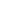#Triangles GEO-1006 is a Course

## Triangles GEO-1006

Self-paced

### Full course description

Course Description

Triangles are polygons that have three sides. You probably see triangles every day in architecture or other areas of life. This course covers the properties of triangles, including right triangles.

By the end of this course, you will be able to

• Classify triangles based on their angles and sides

• Calculate the area of a triangle

• Calculate the lengths of the sides of a right triangle using the Pythagorean theorem

• Understand the proportions of 30o-60o-90o and 45o-45o-90o right triangles

• Calculate the length of a side or the measure of an angle using trigonometric functions

Estimated completion time (hours): 1.3